## Pump Efficiency

The pump efficiency can be evaluated using following efficiency formula: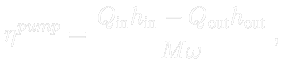(6.3)

where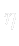denotes the efficiency,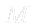is the torque,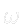is the angular velocity,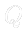is the volume flow rate and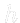is the specific enthalpy which can be evaluated as follows: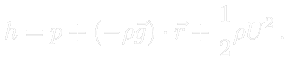(6.4)

Subscriptin means averaged quantities at the inlet, whereas subscriptout denotes averaged quantities at the outlet. The evaluation of pump efficiency for different patches is also possible.